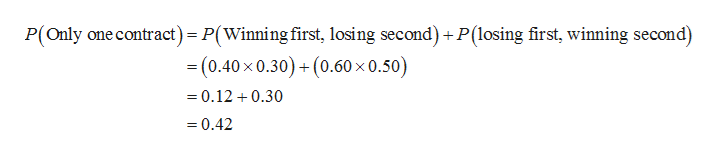# An aerospace company has submitted bids on two separate federal government defense contracts. The company president believes that there is a 40 percent probability of winning the first contract. If they win the first contract, the probability of winning the second contract is 70 percent. However, if they lose the first contract, the president thinks the probability of winning the second contract decreases to 50 percentA.  What is the probability that they win both contracts B.  What is the probability that they lose both contracts C.  What is the probability that they win only one contract Please do not solve using Microsoft Excel.  My professor is not teaching using excel.  I would like to see the steps taken to getting the solution for my own reference to study.

Question
51 views

An aerospace company has submitted bids on two separate federal government defense contracts. The company president believes that there is a 40 percent probability of winning the first contract. If they win the first contract, the probability of winning the second contract is 70 percent. However, if they lose the first contract, the president thinks the probability of winning the second contract decreases to 50 percent

A.  What is the probability that they win both contracts

B.  What is the probability that they lose both contracts

C.  What is the probability that they win only one contract

Please do not solve using Microsoft Excel.  My professor is not teaching using excel.  I would like to see the steps taken to getting the solution for my own reference to study.

check_circle

Step 1

A.

The probability that they win both contracts is obtained below:

The required probability is,

Step 2

B.

The probability that they lose both contracts is obtained below:

The required probability is,

Step 3

C.

The probability that they win only one contract is o...help_outlineImage TranscriptioncloseP(Only one contract) P(Winning first, losing second) +P(losing first, winning second) - (0.40x 0.30)+(0.60 x 0.50) =0.12 0.30 0.42 fullscreen

### Want to see the full answer?

See Solution

#### Want to see this answer and more?

Solutions are written by subject experts who are available 24/7. Questions are typically answered within 1 hour.*

See Solution
*Response times may vary by subject and question.
Tagged in

### Other